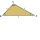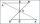# Triangle inequality + reason - math problems

#### Number of problems found: 4

• TrianglesFive sticks with a length of 2,3,4,5,6 cm. How many ways can you choose three sticks to form three sides of a triangle?
• TrianglesHanka cut the 20 cm long straws into three pieces each piece had a length in cm. Then, with these three pieces, she tried to make a triangle. a) What circuit has each of the triangles? b) How long can the longest side measure? c) How many different triang
• TrianglesIvo wants to draw all the triangles whose two sides of which have a length of 4 cm and 9 cm, and the length of the third side is expressed in whole centimeters. How many triangles does he have?
• Right trianglesHow many right triangles we can construct from line segments 3,4,5,6,8,10,12,13,15,17 cm long? (Do not forget to the triangle inequality).

We apologize, but in this category are not a lot of examples.
Do you have an exciting math question or word problem that you can't solve? Ask a question or post a math problem, and we can try to solve it.

We will send a solution to your e-mail address. Solved examples are also published here. Please enter the e-mail correctly and check whether you don't have a full mailbox.

Triangle inequality - math problems. Reason - math problems.